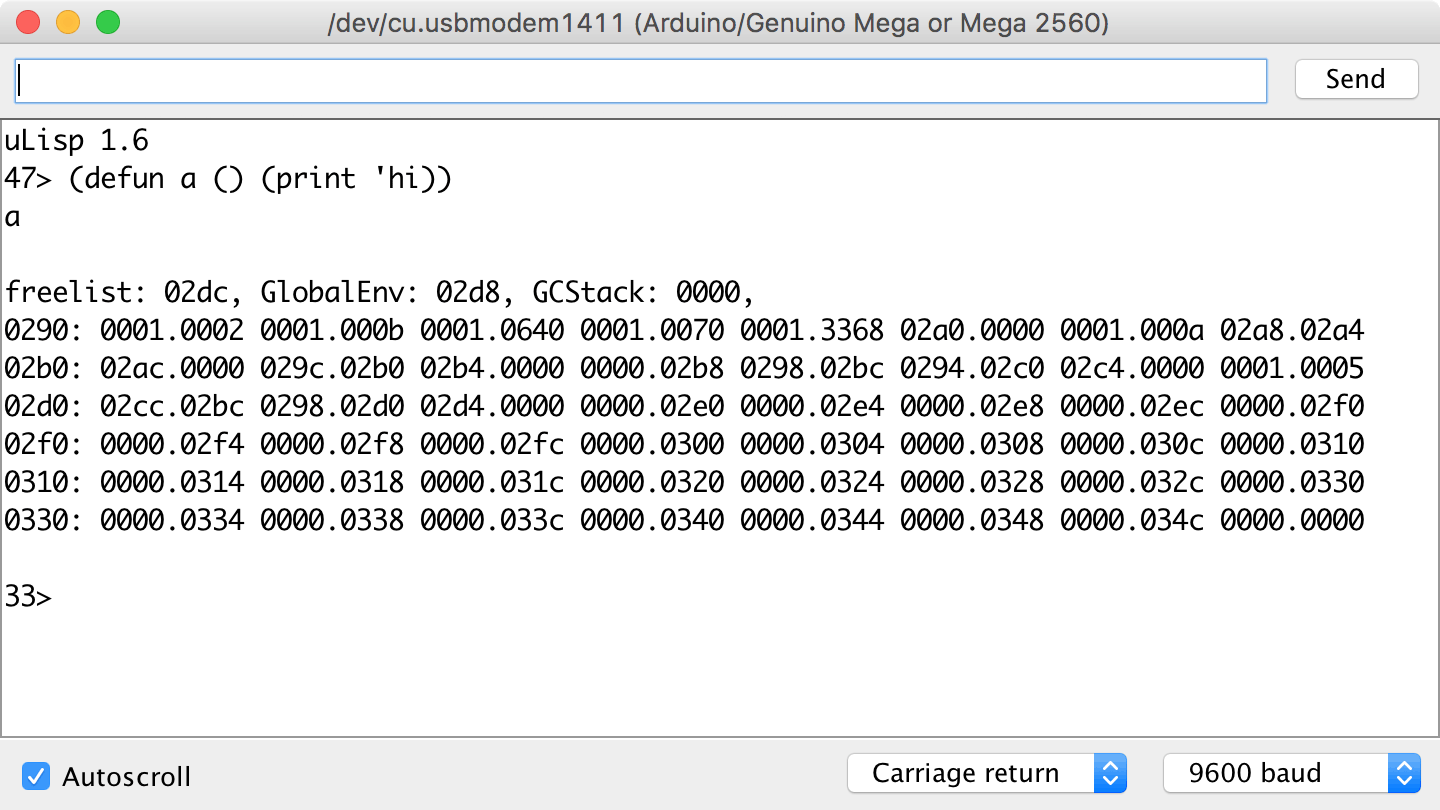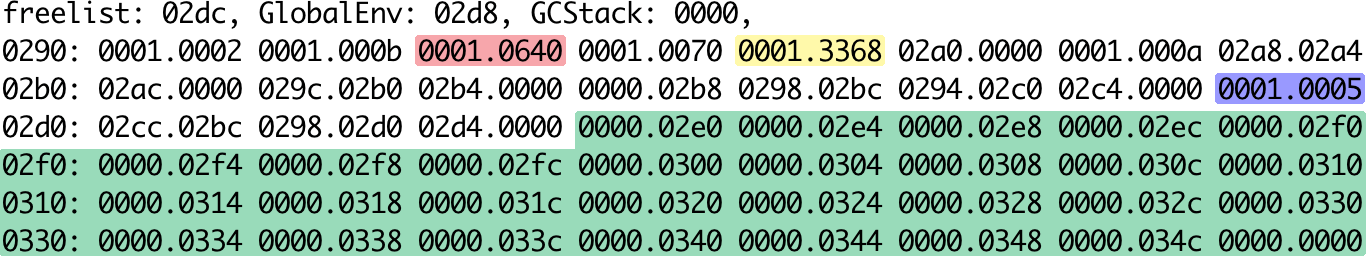## Debugging uLisp

When debugging uLisp it's often useful to be able to dump the uLisp workspace, so you can trace the pointers and check that objects are being represented correctly. In case it's useful to anyone interested in extending uLisp, here's the procedure I use.

First add the function dumpimage() to the source:

```void dumpimage() {
int imagesize = WORKSPACESIZE;
char tmp;
Serial.println();
sprintf(tmp, "Freelist: %04x, ", (int)Freelist);
Serial.print(tmp);
sprintf(tmp, "GlobalEnv: %04x, ", (int)GlobalEnv);
Serial.print(tmp);
sprintf(tmp, "GCStack: %04x, ", (int)GCStack);
Serial.print(tmp);

for (int i=0; i<imagesize; i++) {
if (i%8 == 0) {
Serial.println();
sprintf(tmp, "%04x: ", (int)&Workspace[i]);
Serial.print(tmp);
}
sprintf(tmp, "%04x.%04x ",
(unsigned int)car(&Workspace[i]) , (unsigned int)cdr(&Workspace[i]));
Serial.print(tmp);
}
Serial.println();
}```

This will print the contents of the workspace in hexadecimal in the Serial Monitor, 8 objects per line.

Then reduce the size of the workspace to a suitable multiple of 8 by changing the definition of workspacesize; for example:

`const int Workspacesize = 32;`

Then insert a call to dumpimage() at an appropriate place in the code; for example, near the end of the for(;;) loop in repl().

After evaluating an object you can then examine the dump to see the effect on the workspace. For example, entering:

`(defun a () (print 'hi))`

will produce:The number at the left of each row is the address of the first object in that row; this is followed by eight objects, in hexadecimal.

The following diagram highlights some of the key features of this dump:• Green: The freelist linked-list of free cells.
• Red: The symbol 'a.
• Yellow: The symbol 'hi.
• Blue: The symbol 'lambda.

Starting from GlobalEnv, 02d8, you should be able to trace the list structure of:

`(a . (lambda () (print 'hi))`